### Fitness

Accordingly we need a fitness function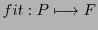with the above mentioned properties. Goldberg proposed the simple function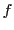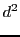(5.11)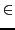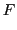(5.12)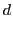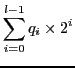(5.13)

Using our population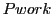from above we can identify the first two elements and translate these binary patterns into decimal numbers with the aid of the scilab function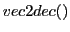from program GA_v4.sce:

-->element1=Pwork(1,:)
element1  =

0.    1.    0.    0.    0.

-->l=5,[d]= vec2dec(element1,l)
l  =

5.
d  =

8.

-->element2=Pwork(2,:)
element2  =

0.    1.    0.    0.    1.

-->l=5,[d]= vec2dec(element2,l)
l  =

5.
d  =

9.


The fitness valuecan then easily be computed. With the possible members in the realm of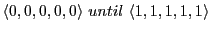we have a possible range of fitness values between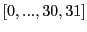. If we assume as a possible thresholdthen we have as set of selected fitness values. Then we get as maximal value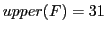. Then we get as possible goal for a genetic process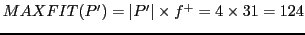. Thus we will have '124' as '100%'.

One simulation of this populationcould then show results (cf. diagram 5.11) where the fitness of the whole population stays below 30% all the time when not using mutation.

Invoking a simulation using the scilab functionfrom the program GA_v4.sce:

-->POP=Pwork, l=5,p=5,n=4,run=100,MThreshold=run,[POP,FITNESS_ALLLOG]=gasimple(POP,l,p,n,run, MThreshold)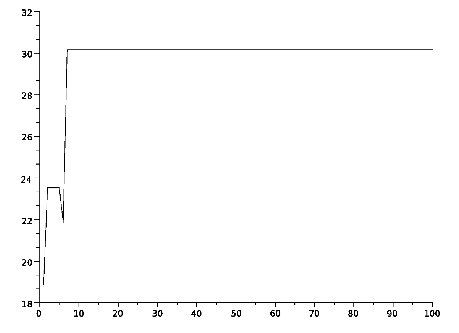Gerd Doeben-Henisch 2013-01-14